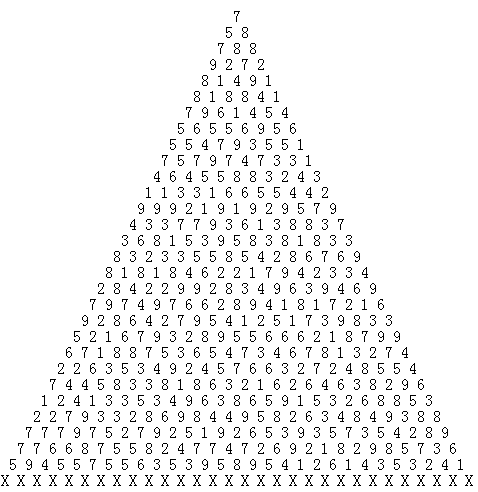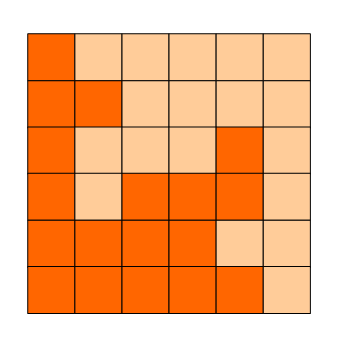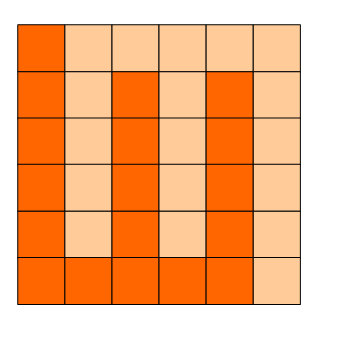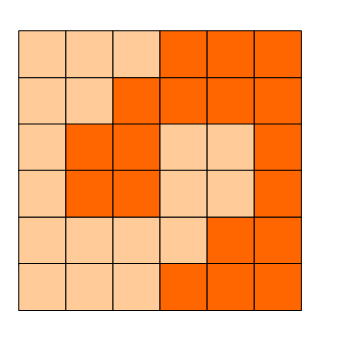打赏

# 2017年第八届蓝桥杯C/C++B组省赛题目解析

## 一、 购物单

-----------------
**** 180.90 88折
**** 10.25 65折
**** 56.14 9折
**** 104.65 9折
**** 100.30 88折
**** 297.15 半价
**** 26.75 65折
**** 130.62 半价
**** 240.28 58折
**** 270.62 8折
**** 115.87 88折
**** 247.34 95折
**** 73.21 9折
**** 101.00 半价
**** 79.54 半价
**** 278.44 7折
**** 199.26 半价
**** 12.97 9折
**** 166.30 78折
**** 125.50 58折
**** 84.98 9折
**** 113.35 68折
**** 166.57 半价
**** 42.56 9折
**** 81.90 95折
**** 131.78 8折
**** 255.89 78折
**** 109.17 9折
**** 146.69 68折
**** 139.33 65折
**** 141.16 78折
**** 154.74 8折
**** 59.42 8折
**** 85.44 68折
**** 293.70 88折
**** 261.79 65折
**** 11.30 88折
**** 268.27 58折
**** 128.29 88折
**** 251.03 8折
**** 208.39 75折
**** 128.88 75折
**** 62.06 9折
**** 225.87 75折
**** 12.89 75折
**** 34.28 75折
**** 62.16 58折
**** 129.12 半价
**** 218.37 半价
**** 289.69 8折
--------------------

#include<stdio.h>
int main()
{
double x,y;
double count=0;
freopen("r1.txt","r",stdin);
while(scanf("%lf%lf",&x,&y)!=EOF)
{
count+=x*y/100;
}
printf("%lf",count);

return 0;
}

## 二、等差素数列

2,3,5,7,11,13,....是素数序列。

2004年，格林与华人陶哲轩合作证明了：存在任意长度的素数等差数列。

#include<iostream>
#include<cstring>
#include<cstdlib>
#include<algorithm>
#include<math.h>
using namespace std;

int main()
{
bool b;
memset(b, 1, sizeof(b));
b = 0;
for (int i = 2; i <= 10000; i++)
for (int j = 2; j <= sqrt(i); j++)
if (!(i % j)) b[i] = 0;
for (int j = 1; j <= 1000; j ++ )
for (int i = 2; i <= 10000 - j * 9; i++)
{
bool yes = 1;
for (int k = 0; k <= 9; k++)
yes = yes && b[i + k * j];
if (yes) {
cout << j;
system("pause");
return 0;
}
}
}

## 三、承压计算

X星球的高科技实验室中整齐地堆放着某批珍贵金属原料。#include<iostream>
#include<fstream>
#include<iomanip>
using namespace std;

int main()
{
fstream in("data.in");
double s, a, b;
memset(s, 0, sizeof(s));

for (int i = 1; i <= 29; i++)
{
for (int j = 1; j <= i; j++)
{
in >> s[i][j];
s[i][j] += (s[i - 1][j] / 2) + (s[i - 1][j - 1] / 2);
}
}
int i = 30;
for (int j = 1; j <= i; j++)
{
in >> s[i][j];
s[i][j] += (s[i - 1][j] / 2) + (s[i - 1][j - 1] / 2);
}
double mi = s, ma=s;
for (int i = 1; i <= 30; i++)
{
if (mi > s[i]) mi = s[i];
if (ma < s[i]) ma = s[i];
}
cout << mi << " " <<(long long)(  ma * (((long long)2086458231) / mi));
system("pause");
return 0;

}

## 四、方格分割

6x6的方格，沿着格子的边线剪开成两部分。#include <algorithm>
#include <string.h>
#include <iostream>
#include <stdio.h>
#include <string>
#include <vector>
#include <queue>
#include <map>
#include <set>
using namespace std;
const int N = 6;
int ans = 0;
int mpt[N+1][N+1];
int dir = {0,1,1,0,0,-1,-1,0};
void dfs(int x,int y)
{
if(x == 0 || y == 0 || x == N || y == N){
ans ++;
return;
}
for(int i = 0 ; i < 4 ; i ++)
{
int tx = x + dir[i];
int ty = y + dir[i];
if(mpt[tx][ty])continue;
mpt[tx][ty] = 1;
mpt[N-tx][N-ty] = 1;
dfs(tx,ty);
mpt[tx][ty] = 0;
mpt[N-tx][N-ty] = 0;
}
}
int main()
{
mpt[N/2][N/2] = 1;
dfs(N/2,N/2);
printf("%d\n",ans/4);
return 0;
}

## 五、取数位

// 求x用10进制表示时的数位长度
int len(int x){
if(x<10) return 1;
return len(x/10)+1;
}

// 取x的第k位数字
int f(int x, int k){
if(len(x)-k==0) return x%10;
return _____________________;  //填空
}

int main()
{
int x = 23574;
printf("%d\n", f(x,3));
return 0;
}#include<stdio.h>

// 求x用10进制表示时的数位长度
int len(int x){
if(x<10) return 1;
return len(x/10)+1;
}

// 取x的第k位数字
int f(int x, int k){
if(len(x)-k==0) return x%10;
return f(x/10,k);  //填空
}

int main()
{
int x = 23574;
printf("%d\n", f(x,3));
return 0;
}

## 六、最大公共子串

#include <stdio.h>
#include <string.h>

#define N 256
int f(const char* s1, const char* s2)
{
int a[N][N];
int len1 = strlen(s1);
int len2 = strlen(s2);
int i,j;

memset(a,0,sizeof(int)*N*N);
int max = 0;
for(i=1; i<=len1; i++){
for(j=1; j<=len2; j++){
if(s1[i-1]==s2[j-1]) {
a[i][j] = __________________________;  //填空
if(a[i][j] > max) max = a[i][j];
}
}
}

return max;
}

int main()
{
return 0;
}#include <stdio.h>
#include <string.h>

#define N 256
int f(const char* s1, const char* s2)
{
int a[N][N];
int len1 = strlen(s1);
int len2 = strlen(s2);
int i,j;

memset(a,0,sizeof(int)*N*N);
int max = 0;
for(i=1; i<=len1; i++){
for(j=1; j<=len2; j++){
if(s1[i-1]==s2[j-1]) {
a[i][j] = a[i-1][j-1]+1;  //填空
if(a[i][j] > max) max = a[i][j];
}
}
}

return max;
}

int main()
{
return 0;
}

## 七、日期问题

----

----

----
02/03/04

----
2002-03-04
2004-02-03
2004-03-02

CPU消耗 < 1000ms

main函数需要返回0;

#include<iostream>
#include<cstring>
#include<cstdlib>
#include<algorithm>
#include<math.h>
#include<string>
using namespace std;
string s;
struct ppp{
long year, mouth, day;
} e;
long tot;
const int od = { 0, 31, 28, 31, 30, 31, 30, 31, 31, 30, 31, 30, 31 };

int get_char(char c)
{
return int(c) - int('0');
}

int cmp(const void  *i, const void *j)
{
struct ppp *a = (struct ppp  *) i;
struct ppp *b = (struct ppp  *) j;
if (a->year != b->year)return a->year > b->year;
if (a->mouth != b->mouth) return a->mouth > b->mouth;
return a->day > b->day;
}

bool pdd(int year, int mouth, int day)
{
int x = 0;
if (!(year % 400) || !(year % 4)) x = 1;
if (mouth > 12) return 0;
if (mouth == 2 && day <= od + x) return 1;
if (day > od[mouth]) return 0;
return 1;
}

void pd(int year, int mouth, int day)
{
if (year < 60) year = 2000 + year;
else year = 1900 + year;
if (pdd(year, mouth, day))
{
++tot;
e[tot].year = year;
e[tot].mouth = mouth;
e[tot].day = day;
}
}

int main()
{
cin >> s;
int x, y, z;
x = get_char(s) * 10 + get_char(s);
y = get_char(s) * 10 + get_char(s);
z = get_char(s) * 10 + get_char(s);
tot = -1;
if (y <= z) swap(y, z);
if (x <= y) swap(x, y);
if (y <= z) swap(y, z);
if (x == y && x == z) pd(x, y, z);
if (x == y && x != z)
{
pd(x, y, z);
pd(z, x, y);
pd(x, z, y);
}
if (z == y && x != z)
{
pd(x, y, z);
pd(z, x, y);
pd(z, y, x);
}
if (x != y && x != z && y != z)
{
pd(x, y, z);   pd(x, z, y);
pd(y, x, z);   pd(y, z, x);
pd(z, x, y);   pd(z, y, x);
}
qsort(e, tot + 1, sizeof(ppp), cmp);
for (int i = 0; i <= tot; i++)
cout << e[i].year << "/" << e[i].mouth << "/" << e[i].day << endl;
system("pause");
}## 八、包子凑数

----

----

2
4
5

6

2
4
6

INF

CPU消耗 < 1000ms

main函数需要返回0;

#include <algorithm>
#include <string.h>
#include <iostream>
#include <stdio.h>
#include <string>
#include <vector>
#include <queue>
#include <map>
#include <set>
using namespace std;
int gcd(int a,int b){
if(b == 0) return a;
return gcd(b,a%b);
}
int arr,n;
const int N = 10010;
bool bk[N];
int main()
{
scanf("%d",&n);
for(int i = 0 ; i < n ; i ++)
scanf("%d",&arr[i]);
int g = arr;
for(int i = 1 ; i < n ; i ++)
g = gcd(g,arr[i]);
if(g != 1)
{
printf("INF\n");
}else{
bk = true;
for(int i = 0 ; i < n ; i ++)
{
for(int j = 0 ; j + arr[i] < N ; j ++)
if(bk[j])bk[j+arr[i]]= true;
}
int count = 0;
for(int i = N-1 ; i >= 0 ; i --){
if(bk[i] == false) count++;
}
printf("%d\n",count);
}
return 0;
}

## 九、分巧克力

1. 形状是正方形，边长是整数
2. 大小相同

2 10
6 5
5 6

2

CPU消耗 < 1000ms

main函数需要返回0;

#include<iostream>
#include<cstring>
#include<cstdlib>
#include<algorithm>
#include<math.h>
#define zy 100002
using namespace std ;
long n , k , h[ zy ] , w[ zy ] , ans ;

bool pd( long  t )
{
long tot = 0 ;
for ( int i = 1 ; i <= n ; i ++ )
tot += ( h[ i ] / t ) * ( w[ i ] / t );
if ( tot >= k ) return 1 ;
return 0 ;
}

void find( long head , long last )
{
if ( head > last ) return ;
long t = (last + head ) >> 1 ;
if ( pd( t ) )
{
ans = max( ans , t ) ;
find( t + 1 , last ) ;
}
else find( head , t - 1 ) ;
}
int main()
{
cin >> n >> k ;
for ( long i = 1 ; i <= n ; i ++ )
cin >> h[ i ]>> w[ i ] ;
ans = 1 ;
find( 1 , zy ) ;
cout << ans ;
return 0 ;
}

## 十、k倍区间

-----

-----

5 2
1
2
3
4
5

6

CPU消耗 < 2000ms

main函数需要返回0;

#include<stdio.h>
long long t={0};
long long a={0};
int main()
{
long long k,n;
scanf("%lld%lld",&n,&k);
int i;
for(i = 1; i<=n;i++)
scanf("%lld",&a[i]);
long long sum=0;

for(i=1;i<=n;i++)
a[i] = (a[i]+a[i-1])%k;
for(i=1;i<=n;i++)
sum+=(t[a[i]]++);

sum+=t;
printf("%lld\n",sum);

return 0;
}

posted @ 2018-03-10 10:59  Zoctopus_Zhang  阅读(...)  评论(...编辑  收藏
// function btn_donateClick() { var DivPopup = document.getElementById('Div_popup'); var DivMasklayer = document.getElementById('div_masklayer'); DivMasklayer.style.display = 'block'; DivPopup.style.display = 'block'; var h = Div_popup.clientHeight; with (Div_popup.style) { marginTop = -h / 2 + 'px'; } } function MasklayerClick() { var masklayer = document.getElementById('div_masklayer'); var divImg = document.getElementById("Div_popup"); masklayer.style.display = "none"; divImg.style.display = "none"; } setTimeout( function () { document.getElementById('div_masklayer').onclick = MasklayerClick; document.getElementById('btn_donate').onclick = btn_donateClick; var a_gzw = document.getElementById("guanzhuwo"); a_gzw.href = "javascript:void(0);"; \$("#guanzhuwo").attr("onclick","follow('33513f9f-ba13-e011-ac81-842b2b196315');"); }, 900);